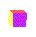# Changing CubesWhat happens to the surface area of a cube when all the dimensions are doubled? tripled?

What happens to the volume of a cube when all the dimensions are doubled? tripled?

If you want to increase the volume of a cube by 216 cubic units, how should you change the dimensions of the cube?

Repeat the investigation above using a rectangular prism and a cylinder.Extension
What happens to the surface area and volume of a rectangular prism and cylinder when only one dimension is modified? How about if two of the dimensions are modified?